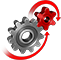# WolframSystemModeler

## Michaelis–Menten Kinetics

A simple enzyme reaction that is not affected by negative feedback, allosteric effects or cooperativity can be simplified using Michaelis–Menten kinetics. This model aims to explain why this is. The free BioChem Modelica library is needed to run this example; it can be downloaded here.

To run this example, you'll need

### Introduction

Michaelis–Menten kinetics is one of the most important models for enzyme-substrate interactions. It is used to study the kinetics in a wide array of biological functions, such as the immune response. While a mass action model of an enzyme-substrate interaction would go through an intermediate step with an enzyme-substrate complex, Michealis–Menten kinetics assumes that this complex rapidly enters quasi steady state and can be approximated. This eliminates the need to measure the formation rate of this complex, which is infeasible in practice.

### The ModelThe mass action model consists of four substances, while the Michaelis–Menten model only consists of two. Still, during certain circumstances, the behavior of the two models is nearly identical.

Visual Representation in SystemModeler

The model has been built in SystemModeler, which results in a visual representation of the model.

The enzyme and the enzyme-substrate complex are implied in the equilibrium description of substrate and product with the help of the Michaelis–Menten rate formula.whereIncorporates All Rate Constants

The Michaelis constant consists of all three reaction rate constants of the regular mass action model, formulated to represent a net reaction flow.,
which is called the Michaelis constant, where,,are the constants for enzyme binding, product formation and substrate unbinding, respectively, as seen below.
E+S### Simulation

The Model Comes to Life

Explore how the rate constants affect the reaction with dynamic sliders.

The model can be explored using the Manipulate functionality in the Wolfram Language. In the downloadable notebook, you can drag the sliders and see how the system responds.Real-time changing of the values of the rate constants allows for experimentation and better understanding of the Michaelis–Menten formula and its associated constants, such asand.

## Wolfram System Modeler

Questions? Comments? Contact a Wolfram expert »